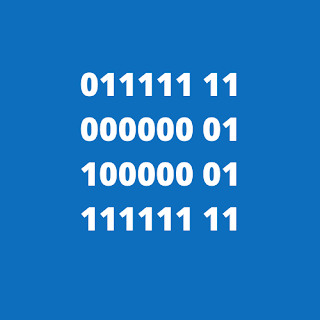Real Numbers Real numbers are defined as the representation of the numbers in the fractional part. While writing down the number in real l...

Real Numbers

Real numbers are defined as the representation of the numbers in the fractional part. While writing down the number in real life, we can use the denary system to write down the real numbers as well. In the case of long numbers, it can be expressed in exponential notation (also known as scientific notation). For example, 43.7 can be expressed as;

.437 x 102 or 4.37 x 101 or 43.7 x 100 or 437 x 10-1

The following example shows the exponential notation of small number which can be expressed in the simple expression but in the case of bigger numbers, a sensible choice would be to use exponential notation.

Floating-point and fixed-point representation

When representing real numbers, binary code (1 & 0) is used for storing the required variables. Fixed-point representation is a method to express the values. While converting the real number into binary codes, the whole number & fractional part need to be defined. Using a defined number of bits for the whole number part and reminder part is needed for overall numbers. Therefore, alternative use is through using floating-point representation. The modern computation uses floating-point representation.

It can be expressed in the following way;

+ M x RE

In this equation, the + M stands for mantissa or significant. Remainder bit of the fractional part in real number is for exponent (E). Whereas, R is not stored, it’s only implicit value of 2.

To show the difference between fixed-point representation and floating-point representation, an example is taken. Let us take, real number to be stored in 8 bits. For the fixed-point option, using a most significant bit a sign bit and next bits for a whole number part leaving two bits for the fractional part.

Some of the largest and smallest values are represented in the table below. The bits implies the position of the binary point.

 Description Binary Code Denary Equivalent Largest Positive Value 011111 11 31.75 Smallest Positive Value 000000 01 0.25 Smallest Magnitude Negative Value 100000 01 -0.25 Largest Magnitude Negative Value 111111 11 -31.75

Table 1.1 (Fixed Point Representation using sign and magnitude)

For using, floating-point representation in the 8-bit real number, four bits is separated for mantissa and four bits is separated for the exponent, using two complement representation.

In this representation, the exponent is stored as a signed integer whereas the mantissa is stored in fixed-point real value.

In the table below, two of the option for the mantissa being expressed in four bits

in each case, the position of binary equivalent points is shown by the gap. These binary equivalent points are defined in The real value in Denary equivalent as shown in the table below.3 largest magnitude positive and negative for integer coding that will be used for the exponent.

 First-bit pattern for real value Real Value in denary 011 1 3.5 011 0 3.0 010 1 2.5 101 0 -3.0 100 1 -3.5 100 0 -4.0

 Second bit pattern for a real value Real value in denary 0 111 .875 0 110 .75 0 101 .625 1 010 -.75 1 001 -.875 1 000 -1.0

 Integer bit pattern An integer value in denary 0111 7 0110 6 0101 5 1010 -6 1001 -8 1000 -8

Table: 1.2 Defining value for a fixed-point representation of the real value in eight bits (four for the mantissa and four for the exponent)

mantissa with an implied binary point immediately follows the sign bit produces smaller spacing between the values that can be represented.

When the mantissa is implied with binary points, the resulted sign is smaller. The space between the values is smaller, thus floating-point representation can be used. Some of the important, non-zero values are presented in the table below. Mantissa and exponent in binary code is distinguished through gap.

 Description Binary Code Denary Equivalent Largest positive value 0 111 0111 .875 x 27 = 112 Smallest positive value 0 001 1000 .125 x 2–8 = 1/2048 Smallest magnitude negative value 1 111 1000 -0.125 x 2-8 = -1/2048 Largest magnitude negative value 1 000 0111 -1 x 27 = -128

Table: 1.3 (Floating-point representation)

In comparison of two tables, 1.1 & 1.2, floating-point representation can be used to express real numbers with a smaller range and larger range values.Name

Chemistry,3,Computer Science,8,Economics,4,General Paper,1,Physics,4,Sociology,4,
ltr
item
Alevel Note: Real Numbers, Floating and fixed point representation
Real Numbers, Floating and fixed point representation
https://1.bp.blogspot.com/-T29JpE0vEvM/X6UcoObzxJI/AAAAAAAAAI4/hpk9u8IqAuIrfmEldKCmELCuLeTlxpmlACLcBGAsYHQ/s320/Black%2Band%2BGrey%2BSpinning%2BCool%2Band%2BFunky%2BComputer%2BLogo.png
https://1.bp.blogspot.com/-T29JpE0vEvM/X6UcoObzxJI/AAAAAAAAAI4/hpk9u8IqAuIrfmEldKCmELCuLeTlxpmlACLcBGAsYHQ/s72-c/Black%2Band%2BGrey%2BSpinning%2BCool%2Band%2BFunky%2BComputer%2BLogo.png
Alevel Note
https://www.alevelnote.com/2020/11/real-numbers-floating-and-fixed-point.html
https://www.alevelnote.com/
https://www.alevelnote.com/
https://www.alevelnote.com/2020/11/real-numbers-floating-and-fixed-point.html
true
1254841511416404733
UTF-8